# Series vs. Parallel Circuit: What’s the Difference?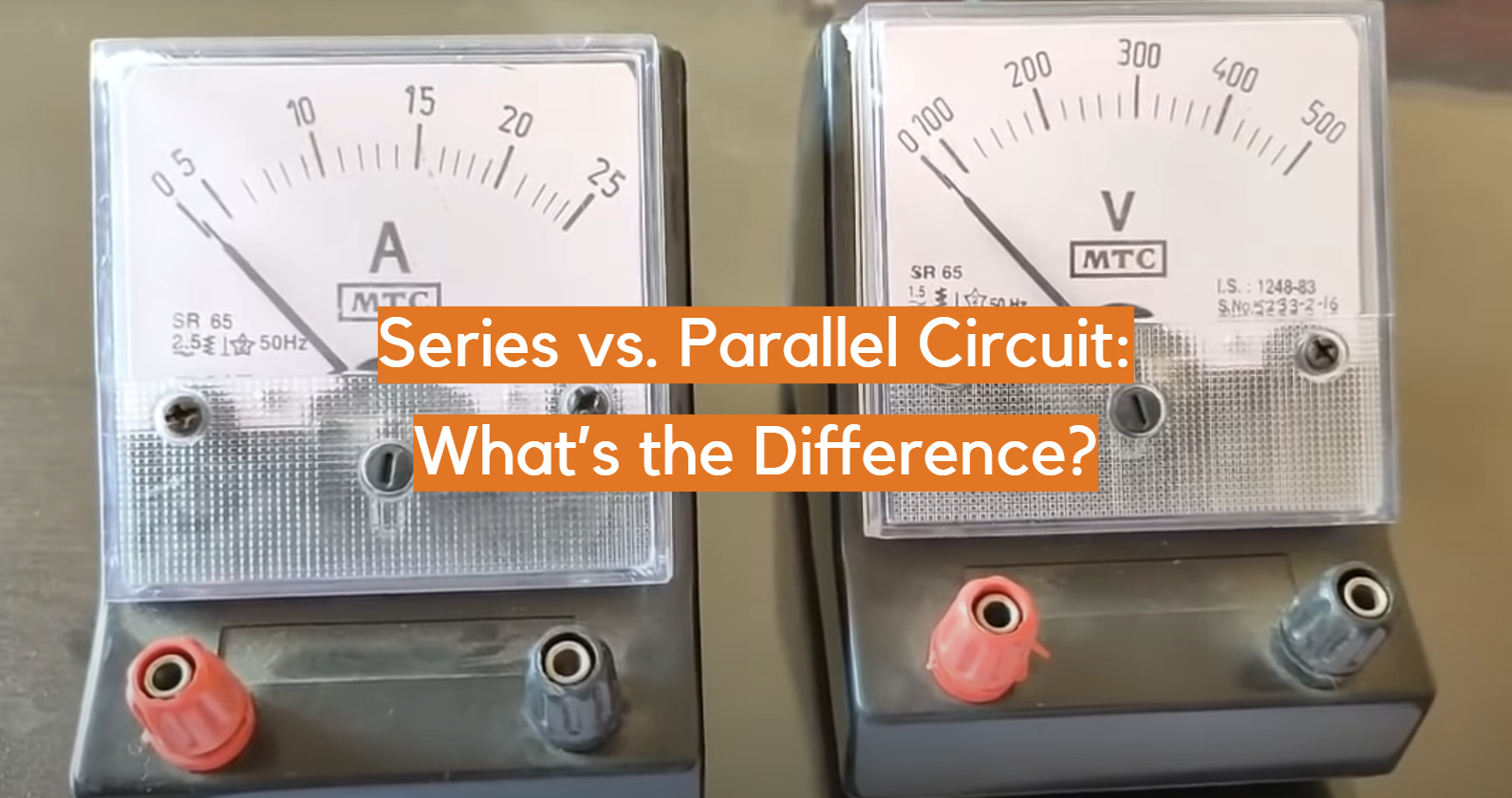If you like working with electricity, it is important to know the basics. Two of the basics are series circuits and parallel circuits.

They are different from each other. Knowing the difference can help you when wiring your projects. If you want to learn more about these types of circuits, now is a good time to start!

In this article, we’ll define what a series vs. parallel circuit is, explore how they’re used differently, and discuss which one might be right for your needs.

So let’s get started- here comes everything you need to know about working with both series and parallel circuits!

## What is a Series Connection?

Series connections are like a line of electrical parts. The electric current flows in one direction, from one part to the next, without going anywhere else. In series circuits, the same amount of voltage goes into each resistor as comes out.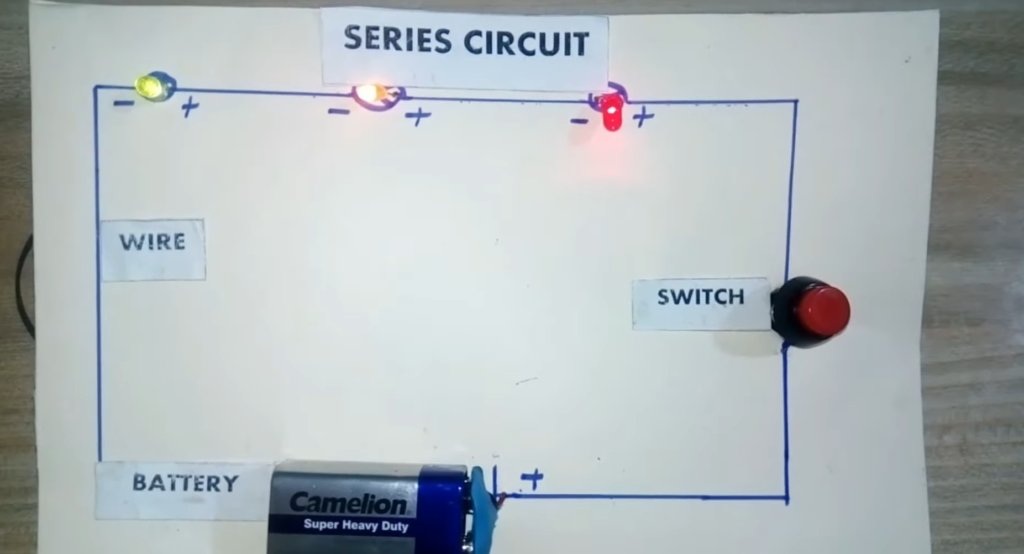### A Series Circuit Example

Let’s say you want to light three LEDs in a row. You would wire them together in series, connecting the negative lead of one LED to the positive lead of the next one in line.

This will ensure that the same amount of current runs through each LED, providing even lighting across all three. 

### What Is a Parallel Connection?

Parallel connections are like branches off a single line.

The electric current can split and go in multiple directions at the same time. The voltage is always shared equally across all of the resistors in a parallel circuit.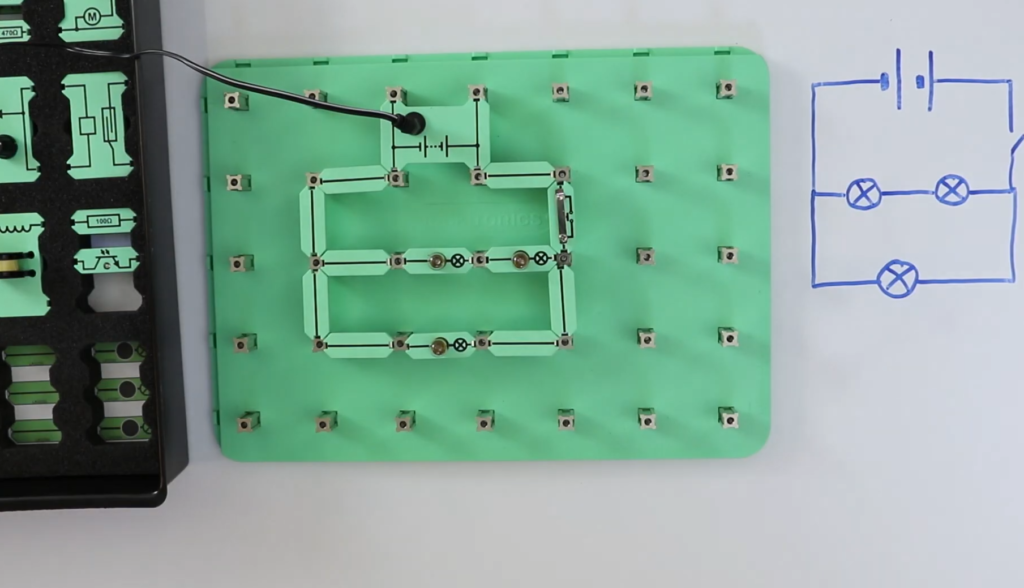### A Parallel Circuit Example

If you want to turn on three LEDs together, don’t put them in a line. Connect the positive lead of one LED to the other two positive leads and the same with the negative leads.

This way, each LED gets an equal amount of power and voltage is shared between all three.

Series and parallel circuits are different. Decide which one is better for your project. Series connections are good for keeping electricity flowing through one line. Parallel connections can power many parts at the same time without affecting each other’s electric current.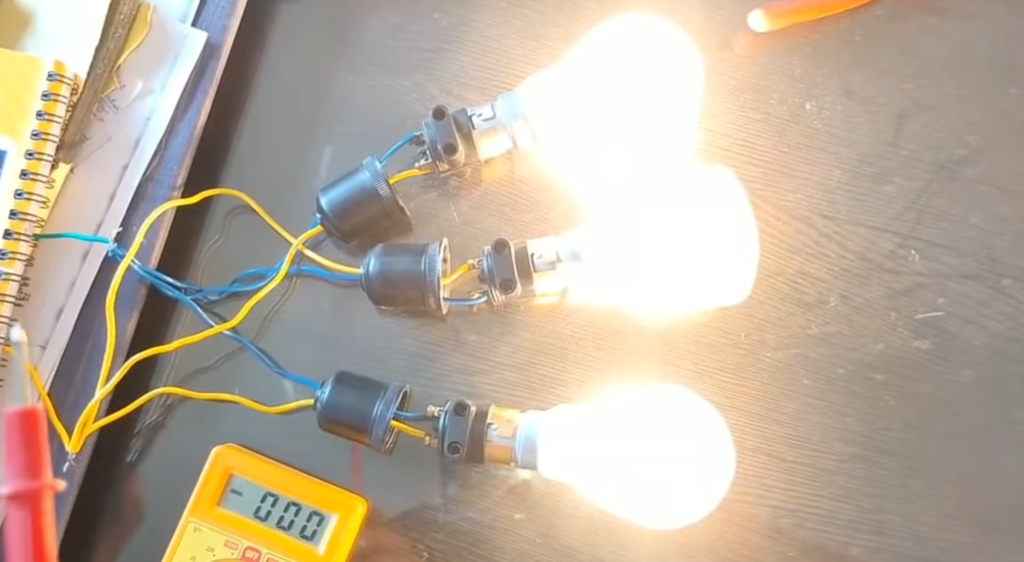## Series vs. Parallel Circuit Review

Series and parallel circuits are different from each other. Series circuits have one continuous flow of electricity, while parallel circuits split the electric current into multiple directions.

Knowing which type to use will help you get the most out of your electrical projects. Consider what is needed for the project, then decide if a series connection or a parallel connection is better suited for your needs.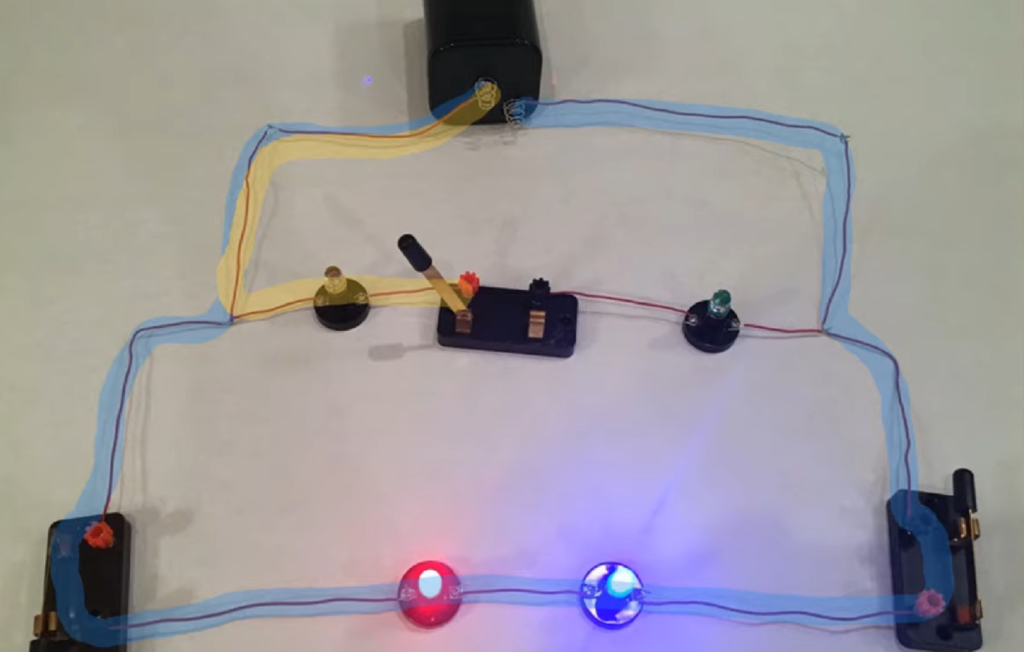## What’s the Difference?

### Voltage

In a series circuit, the voltage stays the same across all components. In a parallel circuit, each component will have its own voltage supplied to it.

### Current

In a series circuit, the current is shared by all components. In a parallel circuit, each component gets its own individual current supply.

### Resistors

In a series circuit, the resistors are connected in line. In a parallel circuit, each resistor is on its own branch.

### Resistance and Conductance in Series Circuits

In series circuits, the total resistance is made up of all the resistors added together. If you add more resistors, it will make the current flow less.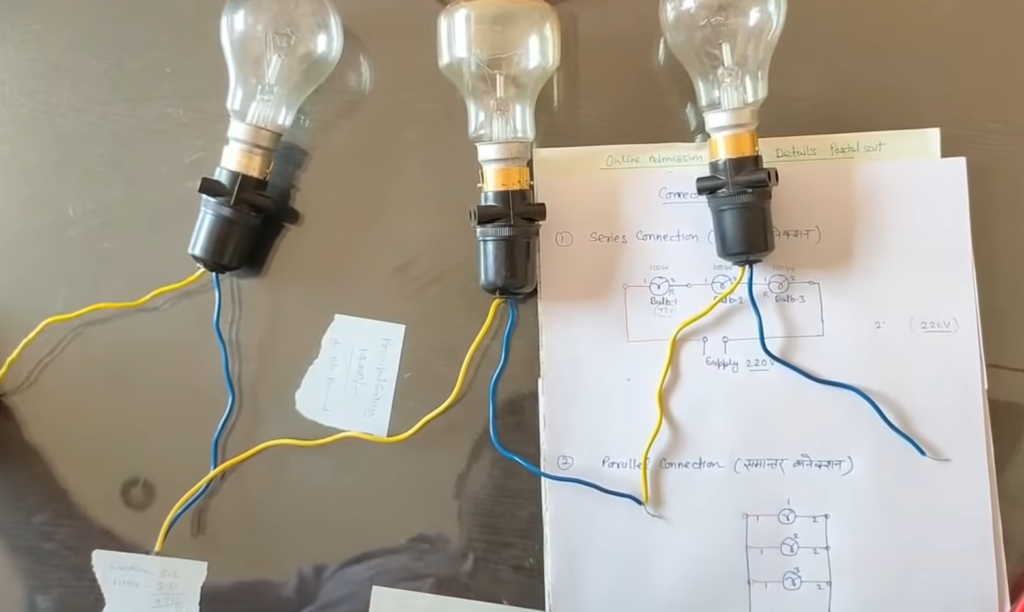In parallel circuits, each resistor has its own value. Adding more resistors does not change the amount of resistance in the circuit and can make current flow more.

### Resistance and Conductance in Parallel Circuits

In parallel circuits, the total resistance is made up of all the resistors in each branch. If you add more resistors to a branch, it will reduce the current flow in that branch.

In series circuits, adding more resistors does not change the amount of resistance and can make current flow less. 

### Switches

In series circuits, the switch will only affect one part of the circuit. In parallel circuits, each branch can be switched on and off independently.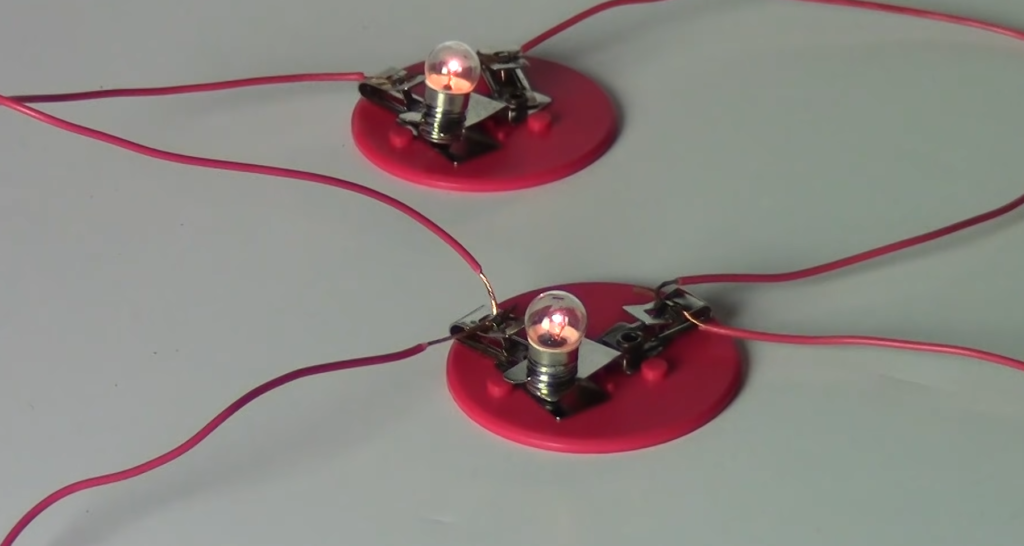## FAQ

### Which is better: parallel or series circuit?

It really depends on your project. If you want the current to flow in one line, use a series circuit. If you want to power multiple parts at the same time without affecting each other, a parallel connection is better suited for your needs.

### How do I know which type of circuit I should use?

Think about what is needed for the project. Consider the voltage and current, as well as the resistance and conductance of each component.

If the circuit is going to stay relatively simple, a series connection may be more suitable. If it’s more complex with multiple parts, a parallel connection may be better.

### What is an example of a series and parallel circuit?

A flashlight is a common example of a series circuit. The battery, bulb and switch are all connected in line, so the current flows through one continuous path.

An LED light strip is an example of a parallel circuit. Each LED has its own positive and negative leads, allowing the electricity to flow in multiple directions at the same time.

### Is voltage the same in series or parallel?

In a series circuit, the voltage is the same across all components. In a parallel circuit, each component gets its own individual voltage supply.

### Does parallel or series have more current?

In a series circuit, the current is shared by all components. In a parallel circuit, each component gets its own individual current supply. Therefore, in general, parallel circuits will have more current than series circuits.

### What are the 3 differences between series and parallel circuits?

Series and parallel circuits are different in three ways. In a series circuit, the voltage is the same for all components. But in a parallel circuit, each component has its own voltage supply.

Also, the current is shared by all components in a series circuit but each component has its own current supply in a parallel circuit.

In a series circuit, the total resistance is made up of all the resistors added together. Extra resistors will make the current flow go down.

In a parallel circuit, each resistor has its own value and adding more will not change the resistance. It can actually help increase current flow.

### Is voltage higher in parallel?

No, voltage is the same in series and parallel circuits. In a parallel circuit, however, each component has its own voltage supply, so the total voltage of the system can be higher if you add more components.

### What is a disadvantage of parallel circuits?

One potential downside of using parallel circuits is that they can be more complex to design and build than series circuits.

Also, because each component has its own current supply, any faults in one branch will not affect the rest of the circuit. This could make it more difficult to diagnose problems.

### What is the relationship between resistance and current in series circuits?

In a series circuit, the total resistance of the circuit is made up of all the resistors added together. If you add more resistors to a branch, it will reduce the amount of current that can flow through it.

This means that if you increase the number of resistors in a series circuit, the amount of current will decrease.

### What is the relationship between resistance and current in parallel circuits?

In a parallel circuit, each resistor has its own value and adding more resistors will not affect the total resistance of the system. It can actually help increase current flow, because it gives more paths for the current to take.

Therefore, in a parallel circuit, adding more resistors will result in an increase of current flow.

### How do you calculate total resistance in a series circuit?

In a series circuit, the total resistance is equal to the sum of all the individual resistors. To calculate it, simply add up all the resistances of each component.

For example, if there are three components with resistances 2Ω, 4Ω and 6Ω respectively, the total resistance of the circuit will be 2+4+6 = 12Ω.

### How do you calculate total resistance in a parallel circuit?

In a parallel circuit, the total resistance of the system is equal to the inverse of the sum of all the individual resistances. To calculate it, take the reciprocal (inverse) of all the resistances and add them up.

For example, if there are three components with resistances 2Ω, 4Ω and 6Ω respectively, the total resistance of the circuit will be 1/2 + 1/4 + 1/6 = 8/12 = 2/3 Ω.

### How does voltage affect current in a circuit?

Voltage is the amount of energy that pushes electricity through a circuit. The higher the voltage, the more current will flow in the circuit.

In other words, if you increase the voltage supply to a circuit, it will cause an increase in current flow. Conversely, decreasing the voltage supply will result in a decrease in current.

### Why is voltage the same in a series circuit?

In a series circuit, all parts are connected in a line. The voltage is the same for each part. It doesn’t change when you add more parts. The total voltage of the system is equal to all of the individual voltages added together.

### What is an advantage of parallel circuits?

One of the main benefits of using a parallel circuit is that it can be used to increase current flow.

Since each component has its own voltage source, adding more components will give electricity more paths to travel through and result in an overall increase of current.

This can be especially useful if you need to power multiple components or devices without sacrificing the amount of current available.

Additionally, because each component has its own power supply, any faults in one branch will not affect the rest of the circuit, making it easier to diagnose and repair problems.

### Does resistance increase or decrease in series circuits?

In a series circuit, the total resistance of the system is made up of all the resistors added together.

This means that if you add more resistors to a branch, it will reduce the amount of current that can flow through it, resulting in an overall increase of resistance.

### Does resistance increase or decrease in parallel circuits?

In a parallel circuit, each resistor has its own value and adding more resistors will not affect the total resistance of the system. In fact, since there are now more paths for electricity to take, it can actually help reduce overall resistance.

This is why increasing the number of components in a parallel circuit usually results in a decrease of resistance.

### Do series circuits have higher or lower current?

In a series circuit, the total amount of current available is fixed and cannot be increased.

This means that each resistor will receive less current than it would if it were connected in a parallel circuit. As such, series circuits usually have lower currents than their parallel counterparts.

### Do parallel circuits have higher or lower current?

In a parallel circuit, the total amount of available current is increased with every additional component.

This is because each component has its own power source and more paths for electricity to travel through. As such, parallel circuits usually have higher currents than their series counterparts.

### Do series circuits have higher or lower voltages?

In a series circuit, the voltage is the same for all components and adding more components will not affect it. Therefore, series circuits usually have lower voltages than their parallel counterparts.

### Which type of circuit is more efficient?

The type of circuit that is most efficient depends on your specific needs. If you need to power multiple components or devices with a high current, then a parallel circuit may be the better choice.

On the other hand, if you are looking for an efficient way to increase the voltage in a system, then a series circuit may be the best option.

Ultimately, it is important to understand the differences between series and parallel circuits in order to choose the one that will work best for your needs.

### Which type of circuit is safer?

Series circuits can be more dangerous because if there is a fault in one component, it will affect the whole system.

In contrast, parallel circuits are generally safer because any faults or problems in one branch will not affect the rest of the circuit. Therefore, parallel circuits may be the better option when safety is a concern.

### Which type of circuit is easier to troubleshoot?

Parallel circuits are usually easier to troubleshoot because any fault or problem in one branch will not affect the rest of the system.

Additionally, it can be easier to identify specific problems when there are multiple paths for electricity to travel through, rather than a single path as with series circuits.

Therefore, if you are looking for an easy way to diagnose and repair problems in your circuit, then a parallel configuration may be the best option.

### Can series and parallel circuits be combined?

Yes, series and parallel circuits can be combined in order to achieve a desired result. For example, you could use a combination of both types of configurations to increase the overall voltage or current output.

It is important to understand how each type of circuit works so that you can create the most efficient system for your needs.

### Can both series and parallel circuits be used in the same circuit?

Yes, both series and parallel circuits can be used within the same system. Combining these two configurations can allow you to achieve a desired result such as increasing voltage or current output.

However, it is important to understand how each type of configuration works and its potential risks before combining them in the same system.

### Can DC and AC be used in the same circuit?

Yes, both DC (direct current) and AC (alternating current) can be used in the same circuit. However, it is important to understand how each type of current works and any potential risks before combining them in the same system.

Additionally, you may need additional components or devices to ensure that the two currents are compatible.

### Can circuit components be swapped out?

Yes, circuit components can be swapped out as long as the old and new components are compatible. This can be done to increase the efficiency of a system or to replace faulty parts.

It is important to understand how each component works and any potential risks before swapping them out in the same system.

Additionally, it may be necessary to adjust other components or devices in the system in order for the circuit to work correctly.

### Is a series circuit always safer than a parallel circuit?

No, a series circuit is not necessarily always safer than a parallel circuit. While it is true that faults or problems in one branch of the parallel configuration will not affect the rest of the system, they can still be dangerous if there are multiple components in the same branch.

Additionally, series circuits can be more dangerous as any fault in one component will affect the whole system.

### Are there any other factors to consider?

Yes, when considering which type of circuit is best for your needs, it is important to take into account the complexity of the system and the intended purpose.

For example, if you are looking for a simple solution then a series circuit may be the better option as it can be easier to troubleshoot.

However, if safety is a concern then a parallel configuration may be the best choice. Additionally, it is important to consider the power requirements of your system and choose components accordingly.

### Which type of circuit is the most efficient?

The most efficient type of circuit will depend on the intended purpose and power requirements. Generally, parallel circuits are considered to be more efficient than series circuits as they can provide better current distribution.

Additionally, combining series and parallel configurations can be an effective way to increase efficiency when dealing with high-power applications.

### Which type of circuit is the most cost-effective?

The most cost-effective type of circuit will depend on the components and power requirements. Generally, a series circuit may be more cost-effective as fewer components are required.

However, a parallel configuration may be better for high-power applications as it can provide better current distribution and an overall increase in efficiency.

Additionally, combining series and parallel configurations can be an effective way to lower the costs of a system without sacrificing performance.

### Is there a way to increase the safety of a circuit?

Yes, there are several ways to increase the safety of a circuit. It is important to choose components that are rated for the intended purpose and power requirements.

Additionally, it is recommended to use surge protectors in high-power applications and consider using fuses or circuit breakers when possible.

## Conclusion

It is important to know the difference between series and parallel circuits when connecting electronic parts to a power source. Series circuits are not too difficult; they take energy from the power source in one direction. But if you connect too many pieces, it can cause problems.

Parallel circuits are better than other types of circuits. They have multiple pathways for electricity. This means lots of things can be connected at once without it getting too much.

Knowing this helps people make the best circuit while making sure they do not create any dangerous conditions or waste electricity.

Be careful when working with series and parallel circuits. Look at the voltage needs of all the parts. Then you can start wiring your circuits safely!